# ISEE Middle Level Math : How to find the solution to an equation

## Example Questions

### Example Question #244 : Algebraic Concepts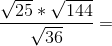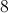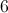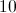Explanation:

First, solve the square roots: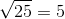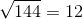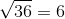Then, solve accordingly: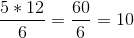### Example Question #41 : How To Find The Solution To An Equation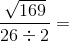Explanation:

First, solve the square root: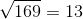Then, solve accordingly: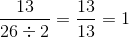### Example Question #41 : Equations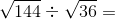Explanation:

First, solve the square roots:Then solve accordingly: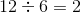### Example Question #42 : How To Find The Solution To An Equation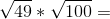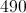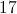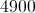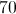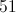Explanation:

First, solve the square roots: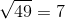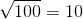Then, solve accordingly: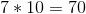### Example Question #1 : How To Solve One Step Equations

A full helium tank can blow up 50 balloons. At least how many times does the tank have to be re-filled in order to blow up 140 balloons?Explanation:

Divide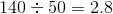Answer: The tank must be filled up at least 3 times.

### Example Question #41 : How To Find The Solution To An Equation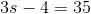What is?Explanation:

First, add 4 to each side: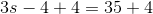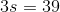Then, divide each side by 3: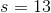### Example Question #42 : How To Find The Solution To An Equation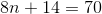What is?Explanation:

First, subtract 14 from each side: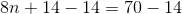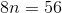Then, divide each side by 8: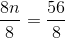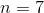### Example Question #43 : Equations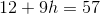What is?Explanation:

First, subtract 12 from each side: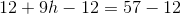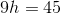Then, divide each side by 9: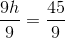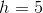### Example Question #1 : Solve Real World And Mathematical Problems By Writing And Solving Equations: Ccss.Math.Content.6.Ee.B.7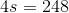What is s?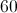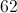Explanation:

Divide both sides by 4: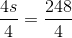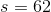### Example Question #45 : Equations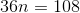Explanation:

First, divide by 36 on each side: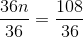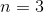### All ISEE Middle Level Math Resources